# Multi-scale Context Aggregation Net Trained onPASCAL VOC2012 Data

Segment an image into various semantic component classes

Released in 2016, this is the first model featuring a systematic use of dilated convolutions for pixel-wise classification. A context aggregation module featuring convolutions with exponentially increasing dilations is appended to a VGG-style front end.

Number of layers: 53 | Parameter count: 141,149,720 | Trained size: 565 MB |

## Training Set Information

• PASCAL VOC2012, a dataset for various computer vision tasks using 20 object classes and 10 action classes.

## Performance

• This model achieves a 73.5% IoU accuracy on the PASCAL VOC2012 dataset.

## Examples

### Resource retrieval

Get the pre-trained net:

 In:=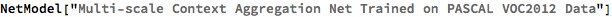Out=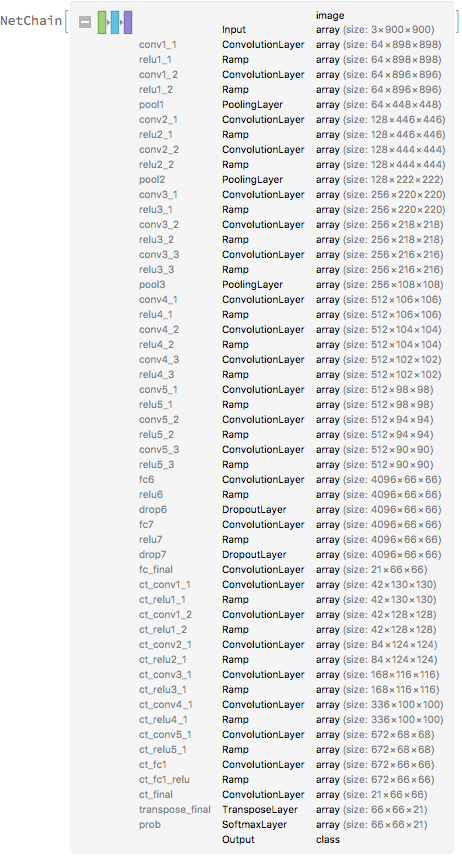### Evaluation function

Write an evaluation function to handle padding and tiling of the input image:

 In:=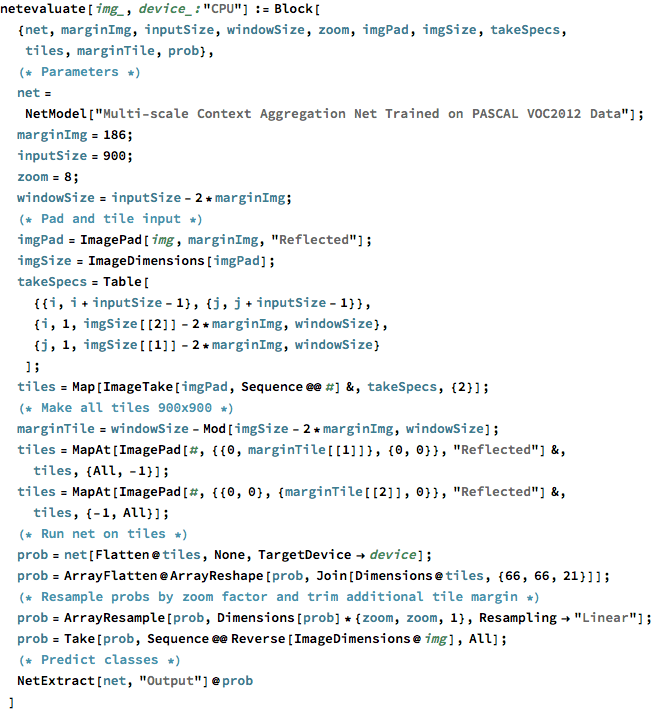### Label list

Define the label list for this model. Integers in the model’s output correspond to elements in the label list:

 In:=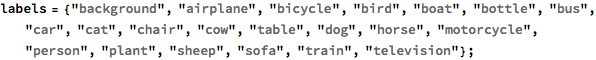### Basic usage

Obtain a segmentation mask for a given image:

 In:=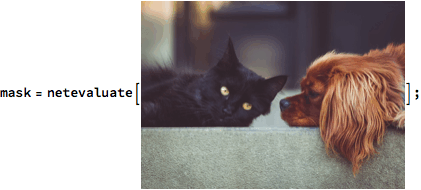Inspect which classes are detected:

 In:=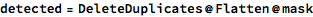Out=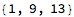In:=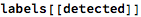Out=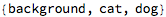In:=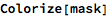Out=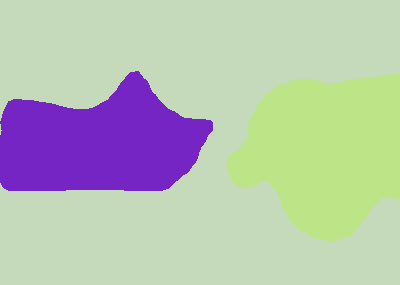Associate classes to colors:

 In:=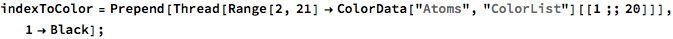Write a function to overlap the image and the mask with a legend:

 In:=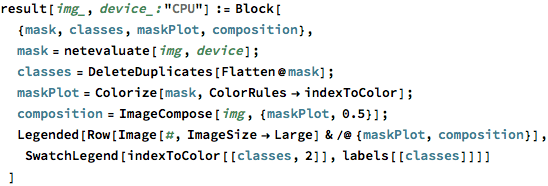Inspect the results:

 In:=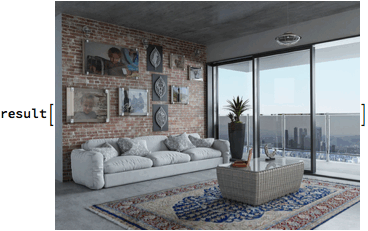Out=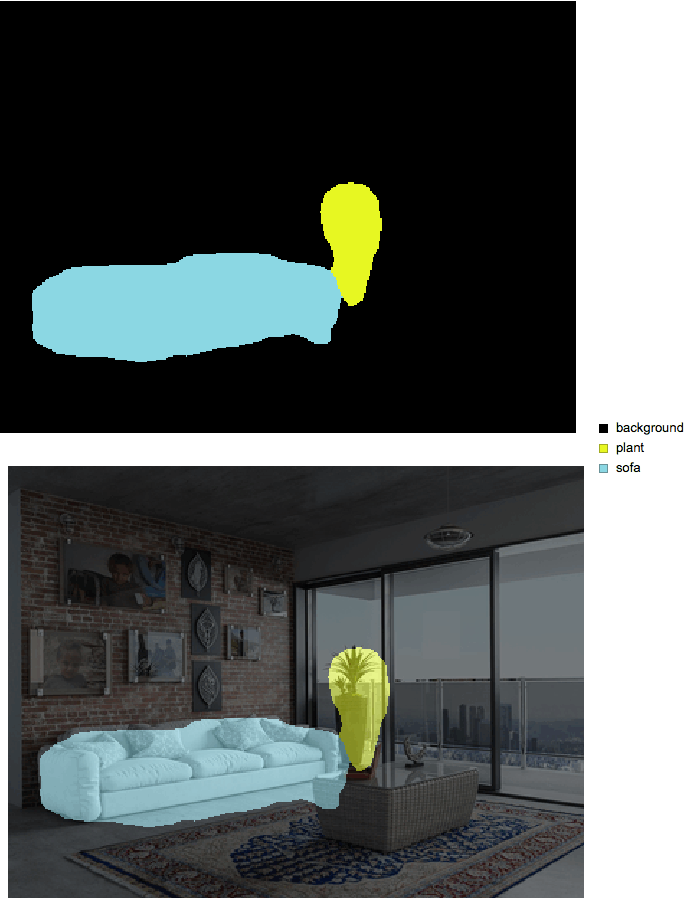In:=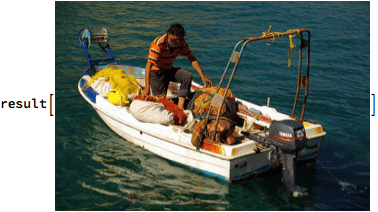Out=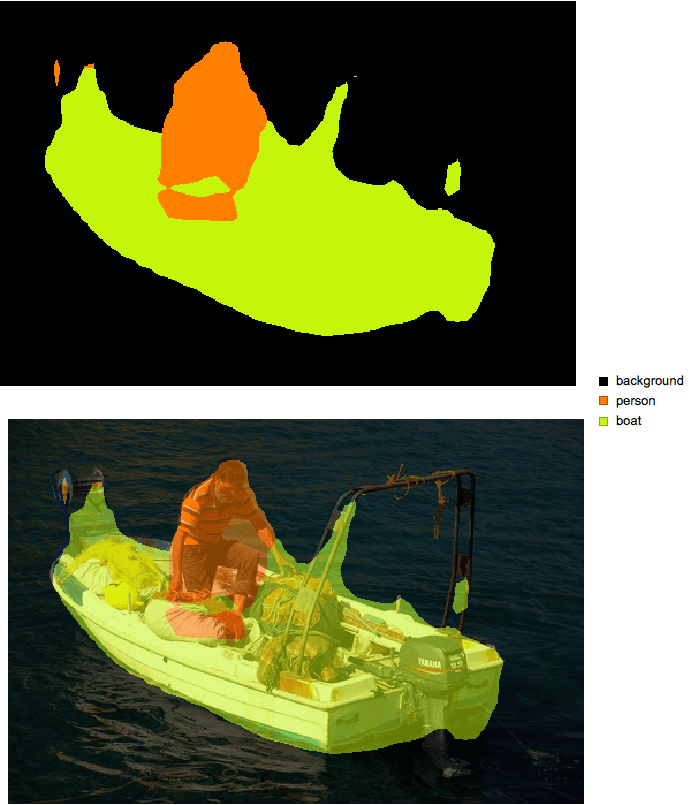In:=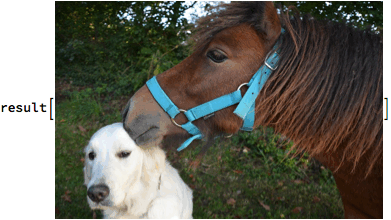Out=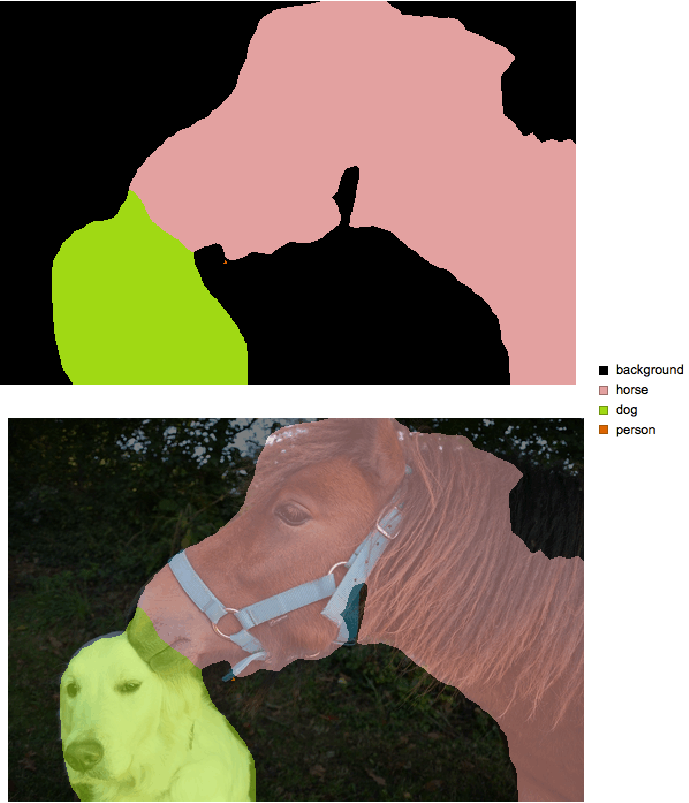### Net information

Inspect the number of parameters of all arrays in the net:

 In:=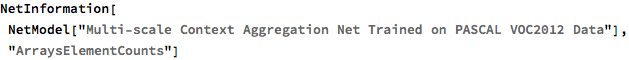Out=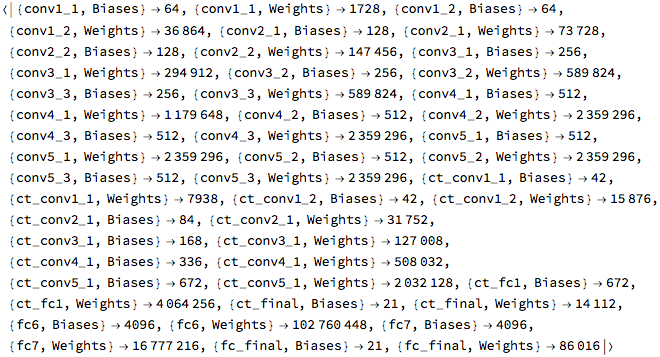Obtain the total number of parameters:

 In:=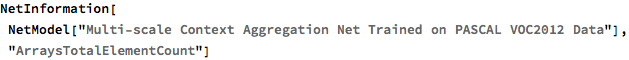Out=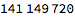Obtain the layer type counts:

 In:=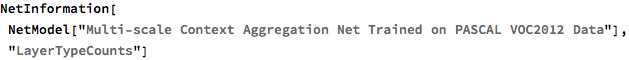Out=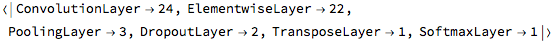### Export to MXNet

Export the net into a format that can be opened in MXNet:

 In:=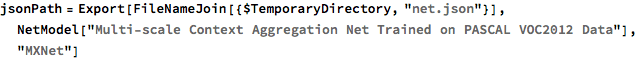Out=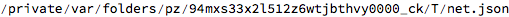Export also creates a net.params file containing parameters:

 In:=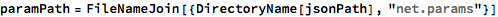Out=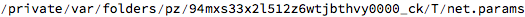Get the size of the parameter file:

 In:=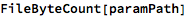Out=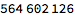The size is similar to the byte count of the resource object:

 In:=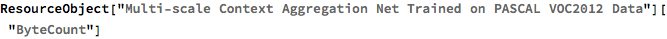Out=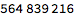Represent the MXNet net as a graph:

 In:=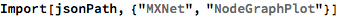Out=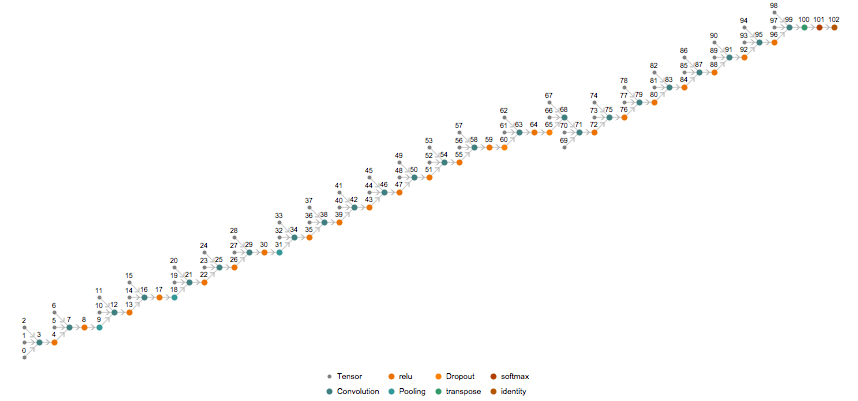## Requirements

Wolfram Language 11.3 (March 2018) or above